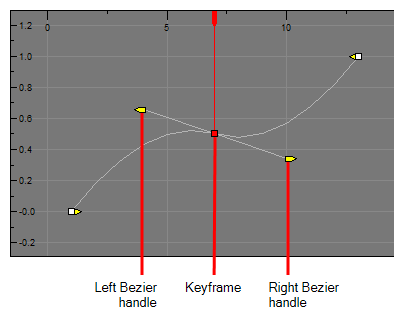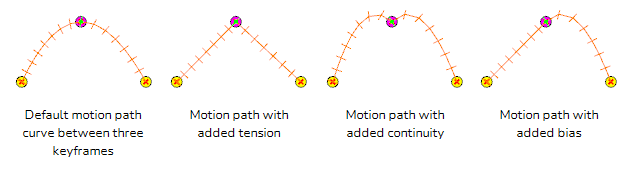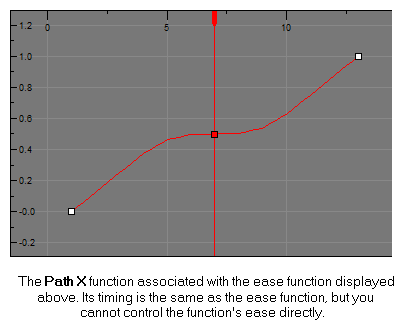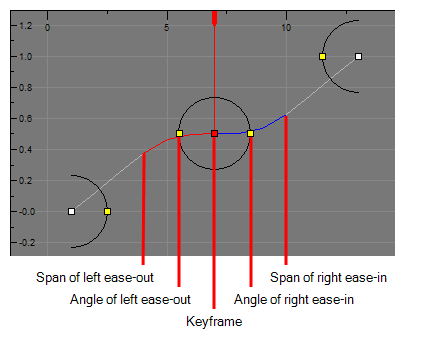# About Animation Functions

T-SBANIM-003-006A

Using the Layer Transform tool, the Timeline view as well as the parameters in the Tool Properties view, you can control the position of each point in a layer, the position of each keyframe in time as well as the ease-in and the ease-out of each movement. Layer animations are composed of functions that can be customized in various other ways. If you need to tweak the animation of a layer in specific ways, you may have to use the Function Editor view.

The Function Editor view displays all the functions which, internally, compose the movement of the selected layer or of the scene's camera. A function is simply a geometric (ie: position, size, angle) component of an animation. When you add a keyframe to a layer or to the camera, a keyframe is created for each animation function for that layer or camera, and that keyframe stores the value for that function at the current frame. Then, the value of the function for each frame between any two keyframes is calculated by Storyboard Pro.

## Animation Functions

A layer or camera animation is composed of the following functions:

• Path X, Y and Z: The position of the object on the x, y and z axes, relative to the centre of the stage. The default value for these functions is 0.
• NOTE In 2D scenes, the Path Z function should always be 0, as it represents the distance of the layer relative to the camera. Changing the value of this function while in a 2D scene will convert the scene to a 3D scene.
• Path Velo: This function is used to adjust the curve of the Path X, Y and Z functions between their keyframes.
• The Path Velo is automatically generated by Storyboard Pro and has one keyframe for each keyframe on the Path X, Y and Z functions. You cannot adjust the value of these keyframes, only the curve between the keyframes. When you do so, the curve between the keyframes of the Path Velo function is applied to the Path X, Y and Z functions. Basically, this allows you to adjust the ease of the object's movement on all three axes simultaneously, using a single function.

In summary, you can edit the Path X, Y and Z function to adjust the position of the object on different keyframes, and the Path Velo function to adjust the pace at which the object moves between keyframes.

NOTE The value of this function always progresses from 0 to 1.
• Scale X, Y and Z: The size of the object on the x, y and z axes relative to its original size. The default value for these functions is 1.
• NOTE The Scale Z function only has a visible effect on 3D objects.
• Angle Z: The rotation angle of the object around the z-axis.
• Quaternion X, Y and Z: If you have rotated the object on any angle but the z-axis, a Quaternion (3D rotation) function is used to track and adjust its angle on each of the x, y and z axes.
• Quaternion Velo: Like the path function, the Quaternion function uses a Velo function to determine the ease of the rotation between keyframes. The values of the keyframes in the Quaternion Velo function cannot be adjusted, only the curve between its keyframes. This curve is applied to the Quaternion X, Y and Z functions, allowing you to adjust the ease of the object's rotation on all three axes simultaneously, using a single function.

## Function types

The functions are of the following types:

• Bezier: A bezier function determines how the interpolation between each keyframe is timed based on Beziers that extend left and right of each keyframe, creating Bezier curves between keyframes.
•• Coordinate: The X, Y or Z component of a 3D Path or 3D Quaternion functions. These functions do not have Beziers nor ease. Their curve is determined by the Velo function to which they are associated (ie: Path Velo or Quaternion Velo).
• For Path functions specifically, each keyframe can have a tension, continuity and bias setting which will influence on how the trajectory of the animation path is curved between each keyframe.Path X, Y and Z functions as well as Quaternion X, Y and Z functions are coordinate functions. However, only Path functions have tension, continuity and bias parameters.• Ease:
• NOTE Ease circles can appear in the Function Editor view if an earlier version of a Storyboard Pro project file is opened in Storyboard Pro 20 or later. If a project file later than Storyboard Pro 20 is opened in Storyboard Pro 7 or earlier, velocity curves will still appear as Bezier. However, if the easing values are modified in the Ease in Ease out boxes, the Bezier editing system changes to the Ease circles customary to earlier versions of Storyboard Pro.

An ease function determines how the interpolation between each keyframe is timed based on the length of the ease, in frames, before and after a keyframe. A function subjected to an ease-in will speed up for the length of the ease-in, then continue at constant pace until it reaches the next keyframe. A function subjected to an ease-out will start out progressing at a constant pace, then start slowing down when the ease-out begins.

Path Velo and Quaternion Velo functions are ease functions.